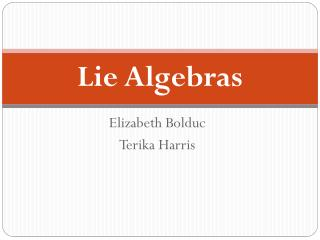DownloadDownload PresentationLie Algebras

# Lie Algebras

Download Presentation## Lie Algebras

- - - - - - - - - - - - - - - - - - - - - - - - - - - E N D - - - - - - - - - - - - - - - - - - - - - - - - - - -
##### Presentation Transcript

1. Lie Algebras Elizabeth Bolduc Terika Harris

2. Lie Algebras: Definition • Let be a vector space over some field, and let and . is a Lie Algebra with a binary operation, known as the Lie Bracket if the following are true: • Bilinear • Alternating • Jacobi identity • Note: Bilinear and Alternating implies anticommutative.

3. A Misconception Marius Sophus Lie • Norwegian Mathematician • Geometry and differential equations

4. Lie Groups • Differentiable manifold • Such that the operations are compatible with the smooth structure. Examples

5. How do Lie Algebras Relate to Lie Groups? • Lie Algebras help us understand Lie Groups. • If G is a Lie Group, the Lie Algebra of G is defined as the tangent space of the identity element of G.

6. Example:

7. Hermann Weyl • Introduced in 1930 • Character Formula • Symmetry

8. Lie Algebras: Definition • Let be a vector space over some field, and let and . is a Lie Algebra with a binary operation, known as the Lie Bracket if the following are true: • Bilinear • Alternating • Jacobi identity • Note: Bilinear and Alternating implies anticommutative.

9. Example • Consider any associative algebra, over some field . • Now we can define our Lie Bracket to be

10. Check that this is a Lie Algebra • Let a, b Bilinear Alternating Jacobi Identity +

11. Example • General Linear Group:

12. Example: Cross Product • with multiplication defined by the cross product • Bilinear? • Alternating? • Jacob Identity?

13. Example Abelian Lie Algebra • If every bracket product is zero • Question: can you name this Abelian Lie Algebra?

14. Lie Algebras: Definition • Let be a vector space over some field, and let and . is a Lie Algebra with a binary operation, known as the Lie Bracket if the following are true: • Bilinear • Alternating • Jacobi identity • Note: Bilinear and Alternating implies anticommutative.

15. Conclusion • Every Lie Group has a corresponding Lie Algebra. • Lie Algebras help us understand Lie Groups